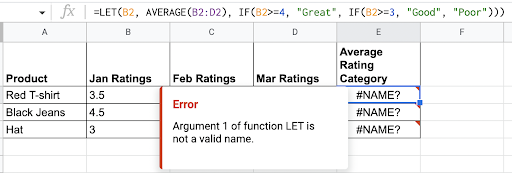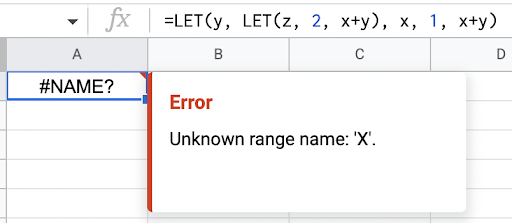# LET function

This function assigns a name with the `value_expression` results and returns the result of the `formula_expression`.

## Sample Usage

`LET(avg, AVERAGE(B2:D2), IF(avg>=4, "Great", IF(avg>=3, "Good", "Poor")))`: Categorize an average value.

`LET(criteria, "Fred", range, FILTER(A2:D8, A2:A8=criteria), ARRAYFORMULA(IF(ISBLANK(range), "-", range)))`: Filter data and replace blank cell with dash.

## Syntax

`LET(name1, value_expression1, [name2, …], [value_expression2, …], formula_expression )`

• `name1`: A name used inside the next `value_expressions` and the `formula_expression`. This must be an identifier (details below), and usage is case-insensitive.
• `value_expression1`: Formula whose result can be referred to later with the name that was declared before. It can use names declared in the previous parameters.
• For example, `AVERAGE(B2:D2)`.
• `name2…`: [ OPTIONAL ] Repeatable, additional `names` to be assigned.
• `value_expression2…`: [ OPTIONAL ] Repeatable, additional `value_expressions` to be evaluated.
• `formula_expression`: Formula to be calculated. It uses `names` declared in the` LET` function.

Tip: You can use `formula_expression` as the `names` defined in the scope of the `LET` function. The `value_expressions` evaluates only once in the `LET` function, even if the next `value_expressions` or the `formula_expression` uses them multiple times.

## Examples

### Categorize the average value of product ratings with LET

Example Data:

A

B

C

D

1

Product

January ratings

February ratings

March ratings

2

Red t-shirt

3.5

4

3

3

Black jeans

4.5

5

3.5

4

Hat

3

2.5

2

With LET: Input this formula in `E2` and drag the blue box around the cell down to fill `E3` and `E4`.

`=LET(avg, AVERAGE(B2:D2), IF(avg>=4, "Great", IF(avg>=3, "Good", "Poor")))`

Without LET: Input this formula in `E2` and drag the blue box around the cell down to fill `E3` and `E4`.

`=IF(AVERAGE(B2:D2)>=4, "Great", IF(AVERAGE(B2:D2)>=3, "Good", "Poor"))`

Result:

A

B

C

D

E

1

Product

January ratings

February ratings

March ratings

Average Rating Category

2

Red t-shirt

3.5

4

3

Good

3

Black jeans

4.5

5

3.5

Great

4

Hat

3

2.5

2

Poor

### Filter data & replace blank cell with dash using LET

Return all data for “Fred” and replace blank cells with dash.

Example Data:

A

B

C

D

1

Rep

Region

Product

Profit

2

Amy

East

Apple

\$1.33

3

Fred

South

Banana

\$0.09

4

Amy

West

Mango

\$1.85

5

Fred

North

\$0.82

6

Fred

West

Banana

\$1.25

7

Amy

East

Apple

\$0.72

8

Fred

North

Mango

\$0.54

With LET: Input this formula in `E2`:

`=LET(criteria, "Fred", range, FILTER(A2:D8, A2:A8=criteria), ARRAYFORMULA(IF(ISBLANK(range), "-", range)))`

Without LET: Input this formula in `E2`:

`=ARRAYFORMULA(IF(ISBLANK(FILTER(A2:D8, A2:A8="Fred")), "-", FILTER(A2:D8, A2:A8="Fred")))`

Result:

E

F

G

H

1

Rep

Region

Product

Profit

2

Fred

South

Banana

0.09

3

Fred

North

-

0.82

4

Fred

West

Banana

1.25

5

Fred

North

Mango

0.54

## Common Errors

The name argument isn’t an identifier

Example: `=LET(B2, AVERAGE(B2:D2), IF(B2>=4, "Great", IF(B2>=3, "Good", "Poor")))`

If the argument isn’t an identifier, this error occurs:Identifier requirements:

• Can’t be ranges, like “A1” or “A2”
• Can’t have spaces or special characters
Left-to-right scoping problem

In a `value_expression` inside the `LET` function, this syntax lets you use the named arguments which have already been declared earlier. For example, “left in the function.”

Example:

 LET(x, 1, y, LET(z, 2, x+z), x+y) CORRECT — x has been declared before using it in the inner LET function. WRONG — using x before its declaration.

If you use an argument before it’s declared, this error occurs:Search
Clear search
Close search